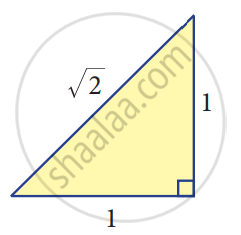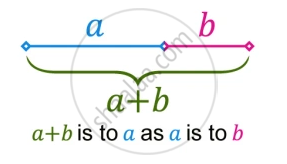# Concept of Irrational Numbers

#### definition

• Irrational numbers: Irrational numbers are numbers that cannot be written in the form p/q, where p and q are integers and q ≠ 0. Example, √2, √3, √15, π, 0.10110111011110.........etc.

# Irrational Numbers:

• Consider an isosceles right-angled triangle whose base and height are each 1 unit long. Using Pythagoras theorem, the hypotenuse can be seen having a length sqrt(1^2 + 1^2) = sqrt2. Greeks found that this sqrt2 is neither a whole number nor an ordinary fraction. The belief of the relationship between points on the number line and all numbers were shattered! sqrt2 was called an irrational number.• Irrational numbers are numbers that cannot be written in the form p/q, where p and q are integers and q ≠ 0.

• Example, √2, √3, √15, π, 0.10110111011110.........etc.

• An irrational number cannot be expressed as a ratio between two numbers and it cannot be written as a simple fraction because there is not a finite number of numbers when written as a decimal. Instead, the numbers in the decimal would go on forever, without repeating.

• ### Examples:

1. Apart from √2, one can produce a number of examples for such irrational numbers. Here are a few: √5, √7, 2√3,......
2. π, the ratio of the circumference of a circle to the diameter of that same circle is another example for an irrational number.
3. e, also known as Euler’s number, is another common irrational number.
4. The golden ratio, also known as the golden mean, or golden section, is a number often stumbled upon when taking the ratios of distances in simple geometric figures such as the pentagon, the pentagram, decagon, and dodecahedron, etc., it is an irrational number.
 GOLDEN RATIO (1:1.6)  The Golden Ratio has been heralded as the most beautiful ratio in art and architecture.Take a line segment and divide it into two smaller segments such that the ratio of the whole line segment (a + b) to segment a is the same as the ratio of segment a to the segment b. This gives the proportion (a + b)/a = a/b. Notice that 'a' is the geometric mean of a + b and b.
If you would like to contribute notes or other learning material, please submit them using the button below.

### Shaalaa.com

Irrational Numbers [00:18:08]
S
0%